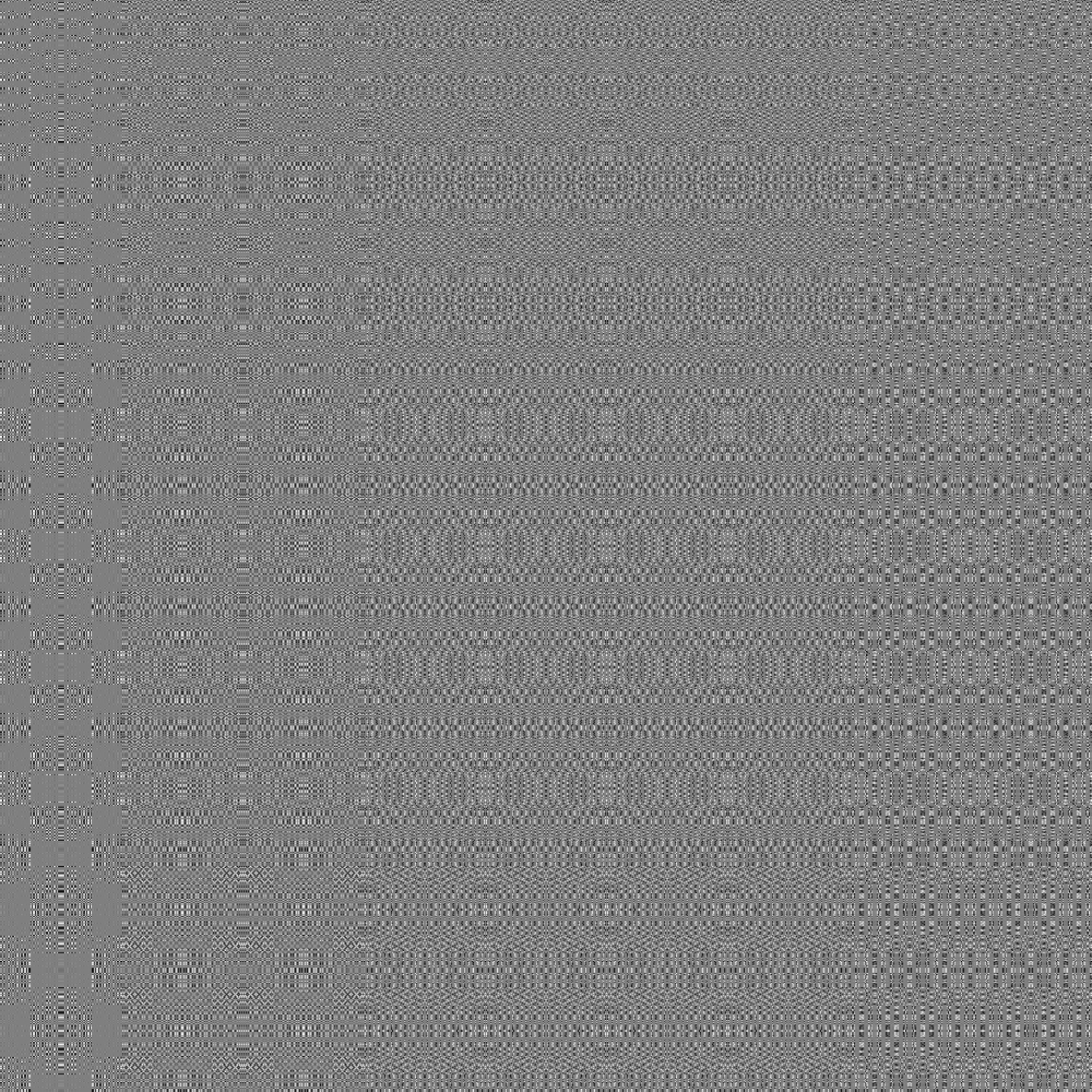White noise is a random noise with equal intensities at different frequencies. It is most well-known as what appeared on old televisions when no signal was found.

noise_white(
dim,
frequency = 0.01,
pertubation = "none",
pertubation_amplitude = 1
)

gen_white(x, y = NULL, z = NULL, t = NULL, frequency = 1, seed = NULL, ...)

## Arguments

dim The dimensions (height, width, (and depth, (and time))) of the noise to be generated. The length determines the dimensionality of the noise. Determines the granularity of the features in the noise. The pertubation to use. Either 'none' (default), 'normal', or 'fractal'. Defines the displacement (warping) of the noise, with 'normal' giving a smooth warping and 'fractal' giving a more eratic warping. The maximal pertubation distance from the origin. Ignored if pertubation = 'none'. Defaults to 1. Coordinates to get noise value from The seed to use for the noise. If NULL a random seed will be used ignored

## Value

For noise_white() a matrix if length(dim) == 2 or an array if length(dim) >= 3. For gen_white() a numeric vector matching the length of the input.

## Examples

# Basic use
noise <- noise_white(c(100, 100))

plot(as.raster(normalise(noise)))# Using the generator
grid <- long_grid(seq(1, 10, length.out = 1000), seq(1, 10, length.out = 1000))
grid$noise <- gen_white(grid$x, grid\$y)
plot(grid, noise)